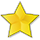BrainDen.com - Brain Teasers

## Question

Write down all the mathematical symbols you know, but exclude any (e.g. cube root) that contain a number. Use as many as you like in as many ways as you like on the arrays of numbers below to make a valid equation. One of them is filled in for you to give an idea for solving the others.

All but 3 - you belong at the Den

All but 1 - you're a free-thinking Denizen

All 10 - Take a bow and a star.0 0 0 = 6

1 1 1 = 6

2 + 2 + 2 = 6

3 3 3 = 6

4 4 4 = 6

5 5 5 = 6

6 6 6 = 6

7 7 7 = 6

8 8 8 = 6

9 9 9 = 6

## Recommended Posts

• 0

(0! + 0! + 0!)! = 6
(1 + 1 + 1)! = 6
2 + 2 + 2 = 6
3 x 3 - 3 = 6
√4 + √4 + √4 = 6
5 + 5/5 = 6
6 + 6 - 6 = 6
7 - 7/7 = 6
8 - √√(8 + 8) = 6
√(9 x 9) - √9 = 6

## Join the conversation

You can post now and register later. If you have an account, sign in now to post with your account.×   Pasted as rich text.   Paste as plain text instead

Only 75 emoji are allowed.

×   Your previous content has been restored.   Clear editor

×   You cannot paste images directly. Upload or insert images from URL.